# Source Association Logic¶

Source association—the process by which individual measurements recorded from an image corresponding to a given time, frequency and Stokes parameter are combined to form a lightcurve representing an astronomical source—is fundamental to the goals of the Transients KSP. However, the process is complex and may be counterintuitive. A thorough understanding is essential both to enable end-users to interpret pipeline results and to inform pipeline design decisions.

Here, we summarise the various possible results of source association, highlighting potential issues. For a full discussion of the algorithms involved, the user is referred to Scheers (2011).

## Database Structure & Association Procedure¶

The structure of the database is discussed in detail elsewhere: here, only a brief overview of the relevant tables is presented.

Each measurement (that is, a set of coordinates, a shape, and a flux) taken is inserted into the extractedsource table. Many such measurements may be taken from a single image, either due to “blind” source finding (that is, automatically attempting to locate islands of significant bright pixels), or by a user-requested fit to a specific position.

The association procedure knits together (“associates”) the measurements in extractedsource which are believed to originate from a single astronomical source. Each such source is given an entry in the runningcatalog table which ties together all of the measurements by means of the assocxtrsource table. Thus, an entry in runningcatalog can be thought of as a reference to the lightcurve of a particular source.

Each lightcurve may be composed of measurements in one or more frequency bands (as defined in the frequencyband table). Within each band flux measurements are collated. These include the average flux of the source in that band, as well as assorted measures of variability. Each row in the runningcatalog_flux table contains flux statistics of this sort for a given band of a given flux. Thus, each row in runningcatalog may be associated with both multiple rows in extractedsource and in runningcatalog_flux. Bear in mind, however, that each lightcurve has a single average position associated with it, stored in the runningcatalog table.

When a new source measurement is made, the association procedure compares it against the records in runningcatalog. Note that the comparison is based upon position only: an association is made if the new measurement is a good fit with a position in runningcatalog, regardless of the time or frequency associated with it. After the association has been made, the position recorded for the source in runningcatalog is updated to take account of the new measurement.

It is important to note that source association must as far as possible be commutative. That is, given a set of measurements, the final contents of the database should not be dependent upon the order of their insertion. This is not possible in the general case—it would involve a quadratic number of source comparisons—but source association procedures should be designed with this goal in mind. In particular, we require that source association be commutative if all measurements made at time $$t_n$$ are inserted and associated before any measurements made at time $$t_{n+1}$$.

### Case Studies¶

Here we will discuss the various outcomes which are possible from the source association process under different conditions. In the following, individual timesteps are indicated by the notation $$t_i$$ and individual flux measurements (that is, at a particular time/band/Stokes) by $$f_j$$. Lightcurves (entries in runningcatalog) are indicated by $$L_k$$; the flux measurements which constitute a particular lightcurve are linked to the $$L_k$$ symbol by means of a coloured line.

#### Single Frequency Band¶

We start by considering observations with only a single frequency band.

##### One-to-One Association¶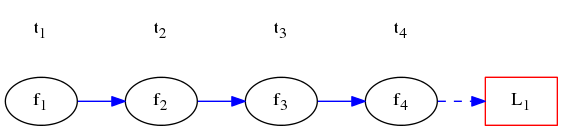In this simplest case all the flux measurements are unambiguously associated in order. A single lightcurve is generated. The calculated average flux of the lightcurve $$L_1$$ is $$\overline{f_{1\cdots{}4}}$$.

##### One-to-Many Association¶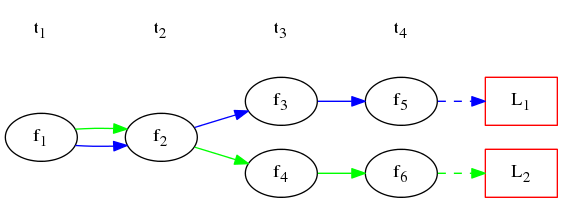Here, both $$f_3$$ and $$f_4$$ can be associated with the lightcurve containing $$f_2$$ and $$f_1$$: a “one-to-many” association. Since $$f_3$$ and $$f_4$$ are distinct, though, they result in two entries in the runningcatalog table, or, equivalently, two lightcurves: $$L_1$$ with average flux $$\overline{f_{1,2,3,5}}$$ and $$L_2$$ with average flux $$\overline{f_{1,2,4,6}}$$.

Note that $$f_1$$ and $$f_2$$: are now being counted twice. Even if $$f_3$$ and $$f_4$$ each contribute only half the total flux of $$f_2$$, the total brightness reached by summing all the lightcurve fluxes increases when this occurs. Equivalently, increasing the spatial resolution of the telescope causes the sky to get brighter!

##### Many-to-One Association¶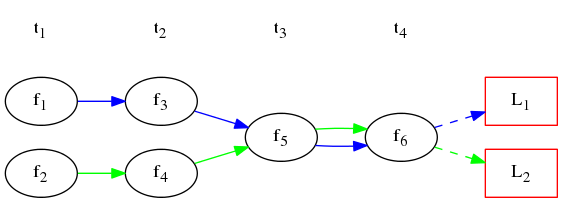This situation is similar to that seen above, but in reverse. Initially, two lightcurves are seen $$L_1$$ consisting of $$f_1$$ and $$f_3$$ and $$L_2$$ consisting of $$f_2$$ and $$f_4$$. However, at timestep $$t_3$$ a new measurement is made, $$f_5$$, which is associated with both $$L_1$$ and $$L_2$$. This, and the subsequent measurement $$f_6$$, are then appended to both lightcurves, resulting in $$L_1$$ having average flux $$\overline{f_{1,3,5,6}}$$ and $$L_2$$ having average flux $$\overline{f_{2,4,5,6}}$$. Again, note that $$f_5$$ and $$f_6$$ are counted twice.

##### Many-to-Many Association¶

Note

First we illustrate “true” many-to-many association. However, for reasons that will become obvious, this is never actually performed: instead, we reduce it to a simpler, one-to-one or one-to-many association.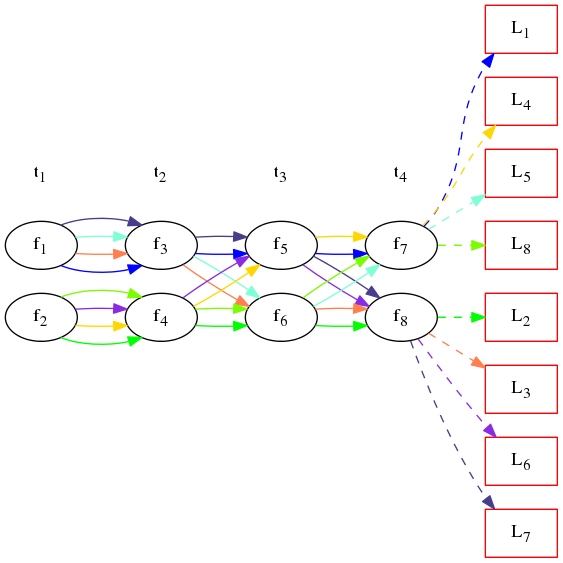As shown above, many-to-many association grows quadratically in complexity, as every possible combination of sources involved in the association results in a new lightcurve. Further, assuming that neither the sky nor the telescope configuration change significantly from observation to observation, it’s likely that subsequent measurements will also result in many-to-many associations, doubling the number of lightcurves at every timestep.

It should be obvious that the scenario described is untenable. Instead, all many-to-many associations are automatically reduced by only taking the source pairs with the smallest De Ruiter radii such that they become either one-to-one or one-to-many associations.

For example, using this criterion, both $$f_5$$ and $$f_6$$ might be associated with a lightcurve consisting of $$f_1$$ and $$f_3$$ in the above. The following situation results: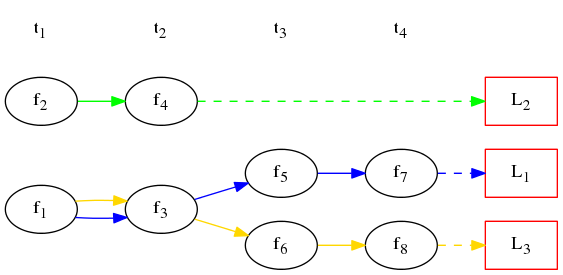Note that $$L_2$$ contains no measurements for timesteps later than $$t_2$$: the many-to-many association is removed, but at the cost of truncating this lightcurve.

#### Multiple Frequency Bands¶

We now introduce the added complexity of multiple bands: the same part of the sky being observed at the same time, but at different frequencies. Here, we use just two bands for illustration, but in practice several could be involved.

When considering multiple frequency bands, the same association procedure, based only on position, as described above, is employed. However, extra care must be taken to ensure that the commutative nature of association is preserved.

##### Multi-Band One-to-One Association¶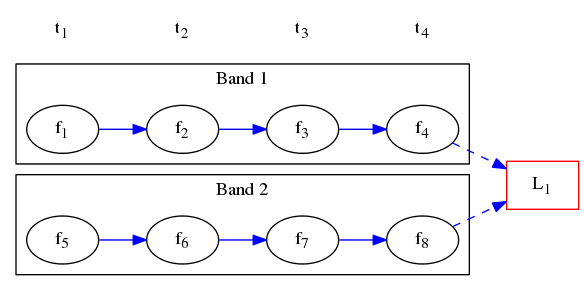In the simplest case, a one-to-one association is made between each measurement and an entry in the runningcatalog table. A single lightcurve results, which we label $$L_1$$, but for which two average fluxes are calculated: $$\overline{f_{1\cdots{}4}}$$ in band 1 and $$\overline{f_{5\cdots{}8}}$$ in band 2.

##### Multi-Band One-to-Many Association¶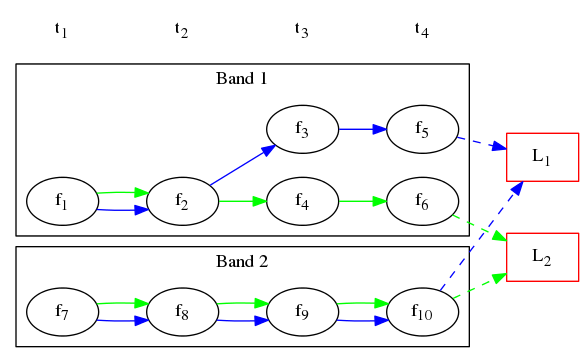Initially, we proceed as above. However, at $$t_3$$, a one-to-many association takes place in Band 1. That band therefore bifurcates, and we are left with two lightcurves: $$L_1$$ and $$L_2$$.

No such bifurcation is seen in Band 2. The single measurement $$f_9$$ may be associated with one or both of $$L_1$$ and $$L_2$$, depending on their relative positions. In the former case, one of the lightcurves is truncated in Band 2. In the latter, a chain of one-to-many associations takes place with measurements in this band, as both $$f_9$$ and $$f_{10}$$ are associated with both lightcurves.

In the situation shown, the resulting average fluxes for $$L_1$$ are $$\overline{f_{1,2,3,5}}$$ in Band 1 and $$\overline{f_{7\cdots{}10}}$$ in Band 2, while those for $$L_2$$ are $$\overline{f_{1,2,4,6}}$$ and $$\overline{f_{7\cdots{}10}}$$ respectively. Note that the entire flux in Band 2, as well as $$f_1$$ and $$f_2$$, is now counted twice.

##### Multi-Band Many-to-One Association¶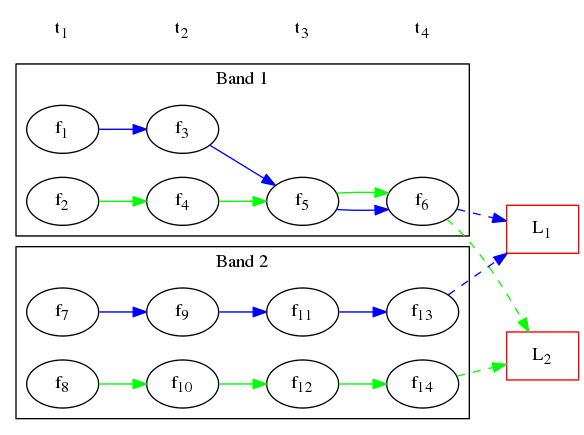At first, $$L_1$$ and $$L_2$$ are completely independent. However, at $$t_3$$, $$f_5$$ undergoes a many-to-one association with both of them. The same applies to $$f_6$$. In Band 2, the lightcurves remain independent. $$L_1$$ therefore has average fluxes $$\overline{f_{1,3,5,6}}$$ in Band 1 and $$\overline{f_{7,9,11,13}}$$ in Band 2, and $$L_2$$ has average fluxes $$\overline{f_{2,4,5,6}}$$ in Band 1 and $$\overline{f_{8,10,12,14}}$$ in Band 2.

##### Multi-Band Many-to-One Association (2)¶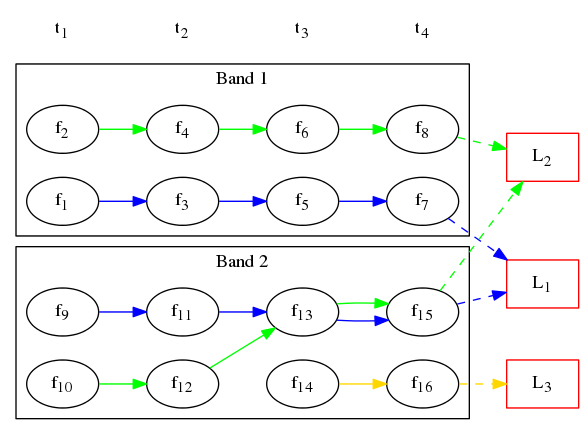In this case, we initially have two separate lightcurves. However, at $$t_3$$, $$f_{13}$$ is associated with both lightcurves in Band 2, while $$f_{14}$$ is associated with neither. Three lightcurves result, as shown.

It is worth considering the ordering of database insertion at this point. In particular, consider that either one of $$f_6$$ and $$f_{14}$$ may be inserted before the other. After each insertion, the average position of the runningcatalog entry is recalculated, and this may affect future associations.

For example, assume that $$f_6$$ is inserted before $$f_{14}$$. In this case, the average position of $$f_{2,4,6,10,12}$$ is not associated with $$f_{14}$$. However, if $$f_{14}$$ were to be inserted first, it would be compared for association with the average position of $$f_{2,4,10,12}$$. This may well produce a different result!

It is desirable for the database contents to be independent of the order of insertion, since this is an arbitrary choice which should not effect scientifically significant outcomes. However, in the current implementation we simply settle for a deterministic and repeatable arbitrary choice, by ingesting images in order of band frequency.

## Discussion¶

It is immediately obvious from the examples given above that, in all but the simplest cases, there is potential for confusion here. In particular, note that simply summing the average fluxes of all the lightcurves in the runningcatalog_flux table in a given band is not an appropriate way to estimate the total brightness of the sky: this may count individual flux measurements multiple times.

Further, the way the source association is handled may result in false detections of transients. In the case of a one-to-many association, for example, a single bright source can be associated with two sources each of a fraction of the brightness. This results in two lightcurves, both containing a (very transient like!) sudden step in flux. A similar outcome can, of course, also result from a many-to-one association.

There are two potential areas of improvement which should be investigated.

Flux division

In a one-to-many or many-to-one association, rather than simply allocating the full flux of the “one” measurement to each of the “many” lightcurves, it could be split such that each was only allotted a portion of the total. In this way, the total brightness of the sky could be maintained.

The most appropriate division is not obvious. A simple model could allocate each of $$n$$ lightcurves a fraction $$1/n$$ of the total flux of the single measurement. A more elaborate procedure would weight the allocation by the flux in each of the $$n$$ lightcurves, such that brighter sources are allocated a larger fraction of the flux.

Whatever flux allocation procedure is adopted, however, involves making assumptions about what fraction should be allocated to each source. Further, it may also increase the computational complexity in the database, as lightcurve statistics are no longer simply calculated over source measurements, but must also take account of fractional allocations.

Smarter association

The current association procedure is purely based on the positions of the sources and their uncertainties. By incorporating more information about the sources, ambiguities in association could often be avoided.

For example, consider the case of a many-to-many association involving an extended source and a point source. It is likely perfectly reasonable to assume that the measurement of the extended source at time $$t_2$$ should only be associated with the extended source at time $$t_1$$, and similarly for the point source: in this way, the many-to-many association can be easily reduced to a much simpler case.

Again, though, a number of assumptions go into any procedure like this. In particular, given that our ultimate aim is to detect transient and variable sources, we should be wary of any procedure that implicitly assumes the sky is unchanging. Further, again the issue of database complexity should be considered: incorporating more logic of this sort is expensive, in terms of both compute and developer time.

## Recommendations¶

Although it is clear that improvements can and will need to be made to the procedures adopted, it is not immediately obvious how best to proceed. Therefore, it is suggested that refinements be deferred until more practical experience has been obtained.

To that end, we suggest the following:

1. Commissioners and scientists working with the lightcurve database, as well as developers of tools designed to detect transients based upon it, must familiarize themselves with the issues described above.
2. The TKP Lightcurve Archive should be explicit about which measurements have gone into a displayed lightcurve or other measurement. The figures which accompany this document are easy to programmatically generate using GraphViz, and show clearly the heritage of a given lightcurve; we suggest, therefore, that they or a derivative of them should be shown on the website.
3. As more source measurements are collected, statistics can be collected to demonstrate to what extent the problems anticipated are observed in real-world use. For example, in the ideal case, the total number of measurements included in all the lightcurves would be equal to the number of measurements made on images; in practice, however, the former will be bigger, since measurements may be counted twice. Observing the “overcounting fraction” as the database grows will help understand the nature and severity of the problem.

## Detailed logic flow¶

Herein we give an algorithmic description of how the source association routines work.

Warning

The following detail is really aimed at developers or particularly interested users only, and can certainly be skipped on first reading.

We assume that source extraction has been run on input images, and new measurements have been inserted into the extractedsource table.

### Clean any previously created temporary listings.¶

To ensure a clean start, we first run _empty_temprunningcatalog, which does what it says on the tin.

### Generate a list of candidate runningcatalog-extractedsource associations¶

This function generates a temporary table listing possible associations with previously catalogued sources.

For a given image_id:

• Select all the relevant extractedsource entries, and
• For each extractedsource, create a bunch of table entries detailing candidate associations with runningcatalog entries which are:
• In the same declination zone as the extractedsource
• Have a weighted mean position for which the RA and DEC are within a box of half-width radius degrees from the extractedsource. (This places a hard limit on the maximum association radius).
• Have a weighted mean position within a user-specified De Ruiter radius of the extractedsource.
• Each of these rows representing a candidate association is populated with all the values which would represent an update to the corresponding runningcatalog and runningcatalog_flux entries, if the association is later determined to be definitive.

### Process all remaining associations¶

Performed by:

We now process all the remaining active associations listed in temprunningcatalog.

_insert_1_to_1_assoc() Inserts all the remaining active links listed in tempruncat, into assocxtrsource. These links all refer to a still-valid runningcatalog entry from a previous source association run. (This actually includes those candidate links in ‘many-to-one’ sets, e.g. sources merged due to a lower-resolution image - hence we set type = 3).

_update_1_to_1_runcat() then performs the corresponding update on the runningcatalog table, copying across the values calculated during the generation of temprunningcatalog.

_update_1_to_1_runcat_flux() grabs all the columns relevant to the runnincatalog_flux entries, from the still active entries in temprunningcatalog, and updates the runningcatalog_flux table accordingly.

### Process remaining extractedsources (those without associations)¶

Performed by:

We still need to insert the ‘new’ sources, i.e. those extractions without an identified association.

_insert_new_runcat() is run first, since the database constraints are already satisfied (pre-existent xtrsrc and dataset-id). First, we pre-select those extractedsources which were discovered in the current image. Then we filter to just those which do not have any associations, by selecting those extractedsources listed in the image but not in the temprunningcatalog (A left outer join on xtrsrc where temprunningcatalog.xtrsrc is NULL).

We initialise the averages (position, flux, etc) by pulling in the relevant values from extractedsource, and the dataset id from the image table.

_insert_new_runcat_flux() performs a similar trick to select the ‘new-source’ extractsources, then cross-matches against the xtrsrc id to select the new runcat entries. With these in hand it’s easy to insert new runcat_flux entries, pulling in the relevant id from runningcatalog, band and stokes from image table, and flux values from extractedsource.

_insert_new_runcat_skyrgn_assocs() performs a positional check against all known skyregions to see which regions this source lies within, and inserts links in the assocskyrgn table accordingly.

_insert_new_assocxtrsource() Performs the same routine of grab ‘new-source’ entries, match new runcat entries, as _insert_new_runcat_flux() - it’s then trival to insert the relevant entries in assocxtrsource. These are then marked as a type = 4 association.

### Cleanup¶

Performed by:

Now that all the new extractions have been dealt with, we take care of some loose ends. We delete all rows from the temprunningcatalog table, and finally delete those runningcatalog entries which we have now superceded, via a simple inactive = TRUE filter.